# Calculus 3 : Normal Vectors

## Example Questions

### Example Question #21 : Normal Vectors

Determine whether the two vectors,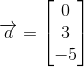and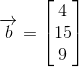, are orthogonal or not.

The two vectors are orthogonal.

The two vectors are not orthogonal.

The two vectors are orthogonal.

Explanation:

Vectors can be said to be orthogonal, that is to say perpendicular or normal, if their dot product amounts to zero: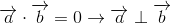To find the dot product of two vectors given the notation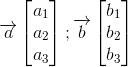Simply multiply terms across rows: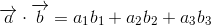For our vectors,and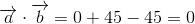The two vectors are orthogonal.

### Example Question #22 : Normal Vectors

Determine whether the two vectors,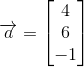and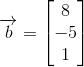, are orthogonal or not.

The two vectors are not orthogonal.

The two vectors are orthogonal.

The two vectors are not orthogonal.

Explanation:

Vectors can be said to be orthogonal, that is to say perpendicular or normal, if their dot product amounts to zero:To find the dot product of two vectors given the notationSimply multiply terms across rows:For our vectors,and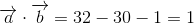The two vectors are not orthogonal.

### Example Question #23 : Normal Vectors

Determine whether the two vectors,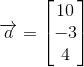and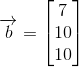, are orthogonal or not.

The two vectors are not orthogonal.

The two vectors are orthogonal.

The two vectors are not orthogonal.

Explanation:

Vectors can be said to be orthogonal, that is to say perpendicular or normal, if their dot product amounts to zero:To find the dot product of two vectors given the notationSimply multiply terms across rows:For our vectors,and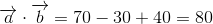The two vectors are not orthogonal.

### Example Question #24 : Normal Vectors

Determine whether the two vectors,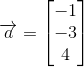and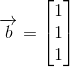, are orthogonal or not.

The two vectors are not orthogonal.

The two vectors are orthogonal.

The two vectors are orthogonal.

Explanation:

Vectors can be said to be orthogonal, that is to say perpendicular or normal, if their dot product amounts to zero:To find the dot product of two vectors given the notationSimply multiply terms across rows:For our vectors,and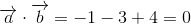The two vectors are orthogonal.

### Example Question #25 : Normal Vectors

Determine whether the two vectors,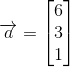and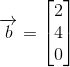, are orthogonal or not.

The two vectors are not orthogonal.

The two vectors are orthogonal.

The two vectors are not orthogonal.

Explanation:

Vectors can be said to be orthogonal, that is to say perpendicular or normal, if their dot product amounts to zero:To find the dot product of two vectors given the notationSimply multiply terms across rows:For our vectors,and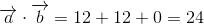The two vectors are not orthogonal.

### Example Question #26 : Normal Vectors

Determine whether the two vectors,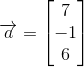and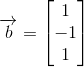, are orthogonal or not.

The two vectors are not orthogonal.

The two vectors are orthogonal.

The two vectors are not orthogonal.

Explanation:

Vectors can be said to be orthogonal, that is to say perpendicular or normal, if their dot product amounts to zero:To find the dot product of two vectors given the notationSimply multiply terms across rows:For our vectors,and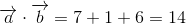The two vectors are not orthogonal.

### Example Question #27 : Normal Vectors

Determine whether the two vectors,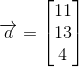and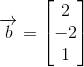, are orthogonal or not.

The two vectors are not orthogonal.

The two vectors are orthogonal.

The two vectors are orthogonal.

Explanation:

Vectors can be said to be orthogonal, that is to say perpendicular or normal, if their dot product amounts to zero:To find the dot product of two vectors given the notationSimply multiply terms across rows:For our vectors,and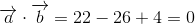The two vectors are orthogonal.

### Example Question #28 : Normal Vectors

Determine whether the two vectors,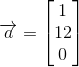and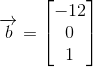, are orthogonal or not.

The two vectors are not orthogonal.

The two vectors are orthogonal.

The two vectors are not orthogonal.

Explanation:

Vectors can be said to be orthogonal, that is to say perpendicular or normal, if their dot product amounts to zero:To find the dot product of two vectors given the notationSimply multiply terms across rows:For our vectors,and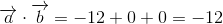The two vectors are not orthogonal.

### Example Question #29 : Normal Vectors

Determine whether the two vectors,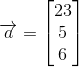and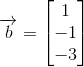, are orthogonal or not.

The two vectors are orthogonal.

The two vectors are not orthogonal.

The two vectors are orthogonal.

Explanation:

Vectors can be said to be orthogonal, that is to say perpendicular or normal, if their dot product amounts to zero:To find the dot product of two vectors given the notationSimply multiply terms across rows:For our vectors,and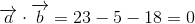The two vectors are orthogonal.

### Example Question #30 : Normal Vectors

Determine whether the two vectors,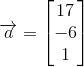and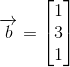, are orthogonal or not.

The two vectors are orthogonal.

The two vectors are not orthogonal.

The two vectors are orthogonal.

Explanation:

Vectors can be said to be orthogonal, that is to say perpendicular or normal, if their dot product amounts to zero:To find the dot product of two vectors given the notationSimply multiply terms across rows:For our vectors,and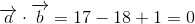The two vectors are orthogonal.